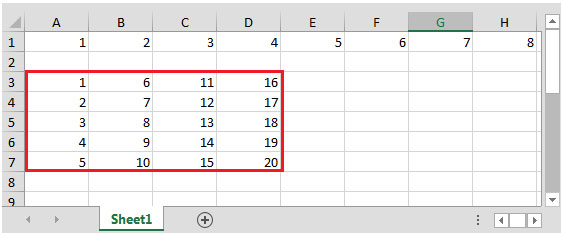# How to Split 1 Row into Multiple Columns in Microsoft Excel 2010

In this article, you will learn how to split 1 row into multiple columns.

If we have a row that contains values & we require copy them into multiple columns meeting the criteria.

We will use a combination of 4 functions i.e. INDEX, MOD, ROWS, COLUMNS

INDEX: Returns a value or reference of the cell at the intersection of a particular row and column, in a given range.

Syntax: =INDEX(array,row_num,column_num)

array: Array is a range of cells or table.

row_num: It is the row number in the array from which to return the value.

column_num: it is optional. It is the column number in the array which is used to return the value.

MOD: Returns the remainder after a number is divided by a divisor.

Syntax =MOD(number,divisor)

number: It is a numeric  value whose remainder you want to find.

divisor: It is the number which is used to divide the number argument.

Rows: It returns the number of rows in a reference or array

Syntax: =(array)

array: Array is a reference to a range of cells or an array.

Columns: Returns the number of columns in a reference or array

Syntax: =(array)

array: Array is a reference to a range of cells or an array.

Let us understand with an example:

Row# 1 contains numbers such as 1,2,3 & so on till 20

Following is a snapshot of the required outputThe criterion is range A3:A7 should contain value stored in cell A1:E1.

After every 5 cells in the first row; the next 5 values (F1:J1) need to be stored in the range B3:B7 & so on.

The formula in cell A3 would be =INDEX(\$1:\$1,,1+MOD(ROWS(\$F\$3:F3)-1,5)+5*(COLUMNS(\$E\$3:E\$3)-1))

Copy down & across the formula, we will get the desired result.

In this way, you can get the values in multiple columns from one row meeting criteria.

1.